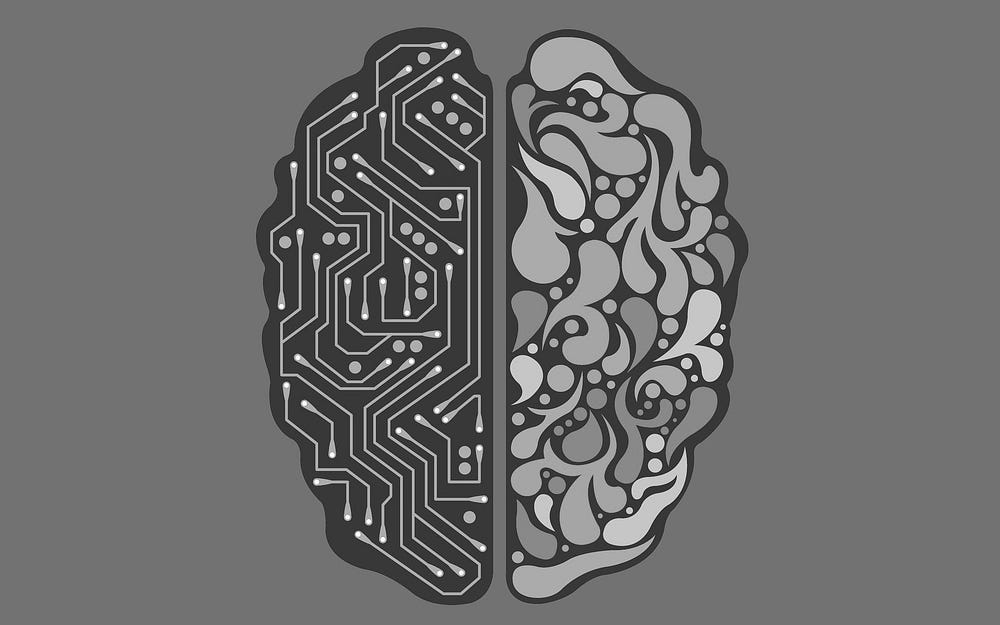Original article was published on Artificial Intelligence on Medium# Neural Networks

neural networks: A biological neural network, made up of actual biological neurons

Neuron: A nerve cell that communicates with other cells via specialized connections

Artiﬁcial neural network: A computing system somewhat inspired by human neural networks, which ‘learns’ to perform tasks without being programmed with task-specific rules and where connections of the neurons are modeled as weights

Step function: A function that increases or decreases abruptly from one constant value to another, for example:

`g(x) = 1 if x ≥ 0, else 0`

Logistic sigmoid: A mathematical function having a characteristic “S”-shaped curve or sigmoid curve, for example:

`g(x) = e[x] / (e[x] +1)`

Rectiﬁed linear unit (ReLU): An activation function, often applied in computer vision, speech recognition & deep neural nets, for example:

`g(x) = max(0, x)`

[For more details, check out Danqing Liu’s Practical Guide to ReLU]

Gradient descent: An algorithm for minimizing loss when training a neural network

Stochastic gradient descent: An iterative method for optimizing an objective function with suitable smoothness properties

Mini-batch gradient descent: A variation of the gradient descent algorithm, splitting the training dataset into small batches, to calculate model error and update model coefficients

Perceptron: A learning algorithm for supervised learning of binary classifiers, or: a single-layer neural network consisting only of input values, weights and biases, net sum, and an activation function

Multilayer neural network: An artificial neural network with an input layer, an output layer, and at least one hidden layer

Backpropagation: An algorithm for training neural networks with hidden layers

Deep neural networks: A neural network with multiple hidden layers

Dropout: Temporarily removing units — selected at random — from a neural network to prevent over-reliance on certain units

Computer vision: Computational methods for analyzing and understanding digital images

Tensorflow: An open-source framework by Google to run machine learning, deep learning, and analytics tasks

[TensorFlow’s previous Medium blog has moved and is now located here…]

Image convolution: Applying a filter that adds each pixel value of an image to its neighbors, weighted according to a kernel matrix

Pooling: Reducing the size of input by sampling from regions in the input

Max-pooling: Pooling by choosing the maximum value in each region

Convolutional neural network: a neural network that uses convolution, usually for analyzing images

Feed-forward neural network: A neural network that has connections only in one direction

Recurrent neural network: A neural network that generates output that feeds back into its own inputs

Now that you’re able to explain the most essential terms around neural networks, you’re ready to follow this rabbit hole further.

Complete your journey to becoming a fully-fledged AI-savvy leader by exploring the other remaining key topics, including Search, Knowledge, Uncertainty, Optimization, Machine Learning, and Language.